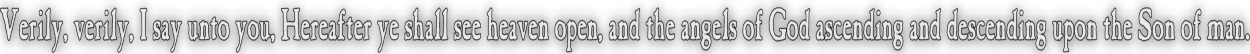None:
Polyps:
Strongs:MetaphysicsFault, Redemption And Salvation We should start with the statement that if we assume the existence of a saving God, we should not make God less for saving a person. We need to put together some simple language. If God indeed has put down some universal code of laws {f} so that those that keep the law might be pleasing to Him, then if an individual x is at fault for breaking the law and incurring a fault on his record (of an "f") then we write f(x). If it is not possible for an "f" to apply to x then ¬P(f(x)) => ¬f(x) i.e. the fault is inapplicable to x and the statement that f is inconsistent or f(x)&¬f(x) is true when applied to x. So we may assume that if x is saved then all faults {f} on x are inconsistent (N¬f(x)). However we require the stronger condition of salvation that implies N(¬f(x)) => f(x)&¬f(x), that f is inconsistent on x even if f(x) and ¬f(x) both make sense. We would asume that f(x) is a negative statement and that ¬f(x) is then a positive statement, but this is not so. ¬f(x) is merely the neutral case, or a lack of privation on perfection as stated by our saving God of his laws {f}. So we must formulate the law that "He whom does these things shall live by them". Fault then is such that the individual may be redeemed by a saving God. But then what is redemption? We may describe redemption to be the possibility that perfection is truly possible in fulfilling every requirement of the law, that no f may apply to some particular x at all. that is, P(¬f(x)) => ¬f(x) (for all f for that x), and that this is not a positive statment If fault is incurred at some time 't1' redemption permits the continuation of a fault f for some interval of time t2-t1, where 't2' is an act of repentance that would redeem the fault. Redemption is therefore the statement that t2=t1 is always possible, and that the application of f(x) at t1 and ¬f(x) at t2 is the statement f(x)&¬f(x), so f is inconsistent for x, and ¬f(x) is no more positive than f(x)&¬f(x). But if f(x)&¬f(x) is not positive, because f is inconsistent for x, we have an idea of that which we mean by "redemption". If an individual takes some interval t2-t1 to return to a state equivalent to redemption, then we state that x is "redeemed." Then we may state that if this is always the case, for any fault f, then "x is saved". Of course, salvation is then the mechanism of God for returning an indixidual x to a state of redemption. If a saving God is simply that, then either we address repentance, or we address salvation. We will see both in the following pages. Continue To Next Page Return To Section Start'